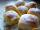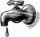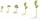Cakes

On the bowl were a few cakes. Jane ate one-third of them, Dana ate a quarter of those cakes that remained.

a) What part (of the original number of cakes) Dana ate?
b) At least how many cakes could be (initially) on thebowl?

Result

a = 1/6b =  6

Solution:Leave us a comment of example and its solution (i.e. if it is still somewhat unclear...):Be the first to comment!To solve this example are needed these knowledge from mathematics:

Need help calculate sum, simplify or multiply fractions? Try our fraction calculator.

Next similar examples:

1. Jam cakesMom baked a third of plum jam cakes, one third cheesecakes and 18 poppy. How many cakes she had bake?
2. Cakes1/3 poppy cake, 1/3 apple, 15 pieces of cheese. How many are totally cakes?
3. ChocolateChildren break chocolate first to third and then every part of another half. What kind got each child? Draw a picture. What part would have received if each piece have halved?Added together and write as decimal number: LXVII + MLXIV
5. Fraction to decimalWrite the fraction 3/22 as a decimal.
6. In fractionsAn ant climbs 2/5 of the pole on the first hour and climbs 1/4 of the pole on the next hour. What part of the pole does the ant climb in two hours?
7. Fraction and a decimalWrite as a fraction and a decimal. One and two plus three and five hundredths
8. Lengths of the poolMiguel swam 6 lengths of the pool. Mat swam 3 times as far as Miguel. Lionel swam 1/3 as far as Miguel. How many lengths did mat swim?
9. ZdeněkZdeněk picked up 15 l of water from a 100-liter full-water barrel. Write a fraction of what part of Zdeněk's water he picked.
10. Mixed2improperWrite the mixed number as an improper fraction. 166 2/3
11. The tapFor one day flows 148 l of water out of the tap. How much water will flow out for 3/4 day?
12. Roses and tulipsAt the florist are 50 tulips and 5 times less roses. How many flowers are in flower shop?
13. Cake 71/3 of a cake shared with 4 people. What share of the whole cake has each people?
14. 3 children3 children had to divide 4 pounds is candy. How much candy did each child get?Add two mixed fractions: 2 4/6 + 1 3/6Of the 72 wet seeds 7/8 germinated. How many seeds germinate?There are 150 pupils in grade 5 . 2/3 of it are female. By what fractions are the males?